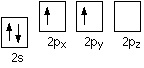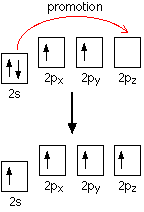How do sp3 orbitals form?

Aug 21, 2016

In short,the linear combinations of the wave functions of s and p orbitals.

Explanation:

Let me use CH4 as an example.
When you do electrons in the box for the carbon you should get thisAs you can see,there are only two bonding pairs of electrons,so carbon in this state can only form 2 bonds(each half filled box represents a potential bond that can be formed).

Remember,when bonds are formed,energy is released,which makes the whole molecule more stable.If carbon could form 4 bonds instead of 2,it would release twice as much energy and be much more stable.

To achieve this,an electron from the 2s orbital is promoted to the empty 2pz orbital. The energy band gap between 2s and 2p is rather small ,so even though it takes energy to promote the electron,the energy released when the 4 bonds form is greater than the energy input required for electron promotion.However,now we have a new problem.We have 4 bonding electrons,but they are in different orbitals,some s and other p,so we won't get 4 identical C-H bonds unless the orbitals are the same.

To give you an analogy,imagine these 4 orbitals in total represent 80 dollars.

The 2s represents 40 dollars

2px represents 16 dollars

2py represents 17 dollars

2pz represents 3 dollars.

If I want to share among the orbitals equally,it would have to be 20 dollars each. To achieve this,I would have to mix the money from all the orbitals ,so each orbital will have 20,with some coming from each.

In the same way if i want 4 equal C-H bonds,the 4 orbitals will have to be the same,so they reorganise themselves into equal orbitals by hybridisation.

Remember ,an orbital can be described by a wave function,so the s and p wave functions combine linearly(the maths of Quantum mechanics is linear).
The 1s orbital is unaffected as the 1s orbitals are too deep inside the atom to be involved in bonding.Basically,inner orbitals are never involved in hybridisation.
Hope this helps.